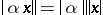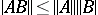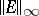# Bauer-Fike theorem

(diff) ← Older revision | Latest revision (diff) | Newer revision → (diff)
Jump to: navigation, search

As popularized in most texts on computational linear algebra or numerical methods, the Bauer–Fike theorem is a theorem on the perturbation of eigenvalues of a diagonalizable matrix. However, it is actually just one theorem out of a small collection of theorems on the localization of eigenvalues within small regions of the complex plane [a1].

A vector norm is a functional $|.|$ on a vector $x$ which satisfies three properties:

1) $| x | | \geq 0$, and $\| x \| = 0$ if and only if $x \equiv 0$.

2)for any scalar $\alpha$.

3) $\| x + y \| \leq \| x \| + \| y \|$ (the triangle inequality). A matrix norm $| A |$ satisfies the above three properties plus one more:

4)(the submultiplicative inequality). An operator norm is a matrix norm derived from a related vector norm:

5) $\| A \| = \operatorname { max } _ { x \neq 0 } \| A x \| / \| x \| = \operatorname { max } _ { \| x \| =1 } \| A x \|$. The most common vector norms are:

\begin{equation*} \| x \| _ { 1 } = \sum _ { i } | x_i |, \end{equation*}

\begin{equation*} \| x \| _ { 2 } = \left( \sum _ { i } | x _ { i } | ^ { 2 } \right) ^ { 1 / 2 } , \| x \| _ { \infty } = \operatorname { max } _ { i } | x _ { i } |, \end{equation*}

where $x_{i}$ denotes the $i$th entry of the vector $x$. The operator norms derived from these are, respectively:

\begin{equation*} \| A \| _ { 1 } = \operatorname { max } _ { i } \sum _ { j } | a _ { i j } |, \end{equation*}

\begin{equation*} \|A\|_{2}= \text{largest singular value of A} ,\, \| A \| _ { \infty } = \operatorname { max } _ { j } \sum _ { i } | a _ { i j} |, \end{equation*}

where $a _ { i j}$ denotes the $( i , j )$th entry of the matrix $A$. In particular, $\| A \| _ { \infty }$ is the "maximum absolute row sum" and $\| A \| _ { 1 }$ is the "maximum absolute column sum" . For details, see, e.g., [a2], Sec. 2.2–2.3.

Below, the notation $|.|$ will denote a vector norm when applied to a vector and an operator matrix norm when applied to a matrix.

Let $A$ be a real or complex $( n \times n )$-matrix that is diagonalizable (i.e. it has a complete set of eigenvectors $v _ { 1 } , \dots , v _ { n }$ corresponding to $n$ eigenvalues $\lambda _ { 1 } , \dots , \lambda _ { n }$, which need not be distinct; cf. also Eigen vector; Eigen value; Complete set). Its eigenvectors make up an $( n \times n )$-matrix $V$; let $\Lambda = \operatorname { diag } \{ \lambda _ { 1 } , \ldots , \lambda _ { n } \}$ be the diagonal matrix. Then $A = V \Lambda V ^ { - 1 }$. Let $E$ be an arbitrary $( n \times n )$-matrix. The popular Bauer–Fike theorem states that for such $A$ and $E$, every eigenvalue $\mu$ of the matrix $A + E$ satisfies the inequality

\begin{equation} \tag{a1} | \mu - \lambda | \leq \| V \| . \| V ^ { - 1 } \| . \| E \|, \end{equation}

where $\lambda$ is some arbitrary eigenvalue of $A$.

If $| E |$ is small, this theorem states that every eigenvalue of $A + E$ is close to some eigenvalue of $A$, and that the distance between eigenvalues of $A + E$ and eigenvalues of $A$ varies linearly with the perturbation $E$. But this is not an asymptotic result valid only as $E \rightarrow 0$; this result holds for any $E$. In this sense, it is a very powerful localization theorem for eigenvalues.

As an illustration, consider the following situation. Let $B$ be an arbitrary $( n \times n )$-matrix, let $A = \operatorname { diag } \{ b _ { 11 } , \dots , b _ { n n } \}$ be the diagonal part of $B$, and define $E = B - A$ to be the matrix of all off-diagonal entries. Let $\mu$ be an arbitrary eigenvalue of $B$. The matrix of eigenvectors for $A$ is just the identity matrix, so the Bauer–Fike theorem implies that $| \mu - b _ { i i } | \leq \| E \|$, for some diagonal entry $b _ {ii }$. The $\infty$-normis just the maximum absolute row sum over the off-diagonal entries of $B$. In this norm the assertion means that every eigenvalue of $B$ lies in a circle centred at a diagonal entry of $B$ with radius equal to the maximum absolute row sum over the off-diagonal entries. This is a weak form of a Gershgorin-type theorem, which localizes the eigenvalues to circles centred at the diagonal entries, but the radius here is not as tight as in the true Gershgorin theory [a2], sec. 7.2.1, (cf. also Gershgorin theorem).

Another simple consequence of the Bauer–Fike theorem applies to symmetric, Hermitian or normal matrices (cf. also Symmetric matrix; Normal matrix; Hermitian matrix). If $A$ is symmetric, Hermitian or normal, then the matrix $V$ of eigenvectors can be made unitary (orthogonal if real; cf. also Unitary matrix), which means that $V ^ { \text{H} } V = I$, where $V ^ { \text{H} }$ denotes the complex conjugate transpose of $V$. Then $\| V \| _ { 2 } = \| V ^ { - 1 } \| _ { 2 } = 1$, and (a1) reduces to $| \mu - \lambda | < \| E \|$. Hence, for symmetric, Hermitian or normal matrices, the change to any eigenvalue is no larger than the norm of the change to the matrix itself.

The Bauer–Fike theorem as stated above has some limitations. The first is that it only applies to diagonalizable matrices. The second is that it says nothing about the correspondence between an eigenvalue $\mu$ of the perturbed matrix $A + E$ and the eigenvalues of the unperturbed matrix $A$. In particular, as $E$ increases from zero, it is difficult to predict how each eigenvalue of $A + E$ will move, and there is no way to predict which eigenvalue of the original $A$ corresponds to any particular eigenvalue $\mu$ of $A + E$, except in certain special cases such as symmetric or Hermitian matrices.

However, in their original paper [a1], F.L. Bauer and C.T. Fike presented several more general results to relieve the limitation to diagonalizable matrices. The most important of these is the following: Let $A$ be an arbitrary $( n \times n )$-matrix, and let $\mu$ denote any eigenvalue of $A + E$. Then either $\mu$ is also an eigenvalue of $A$ or

\begin{equation} \tag{a2} 1 \leq \| ( \mu I - A ) ^ { - 1 } \cdot E \| \leq \| ( \mu I - A ) ^ { - 1 } \| \cdot \| E \| . \end{equation}

This result follows from a simple matrix manipulation, using the norm properties given above:

\begin{equation*} ( A + E ) x = \mu x = ( \mu I ) x \Rightarrow \end{equation*}

\begin{equation*} \Rightarrow ( \mu I - A ) ^ { - 1 } . E x = x \Rightarrow \Rightarrow \| ( \mu I - A ) ^ { - 1 } . E \| . \| x \| \geq \| x \|. \end{equation*}

Even though this may appear to be a rather technical result, it actually leads to a great many often-used results. For example, if $A = V \Lambda V ^ { - 1 }$ is diagonalizable, it is easy to show that

\begin{equation*} \| ( \mu I - A ) ^ { - 1 } \| = \| V ( \mu I - A ) ^ { - 1 } V ^ { - 1 } \| \leq \end{equation*}

\begin{equation*} \leq \| V \| \cdot \| ( \mu I - A ) ^ { - 1 } \| \cdot \| V ^ { - 1 } \|, \end{equation*}

which leads immediately to the popular Bauer–Fike theorem (using the fact that the operator norm of a diagonal matrix is just its largest entry in absolute value). One can also repeat the construction, in which $B$ is an arbitrary matrix, $A$ is the matrix consisting of the diagonal entries of $B$, and $E = B - A$ is the matrix of off- diagonal entries. Using the norm $\| . \| _ { \infty }$, the leftmost inequality in (a2) then reduces to

\begin{equation*} 1 \leq \operatorname { max } _ { i } \left( \frac { 1 } { | \mu - b _ { i i } | } . \sum _ { j \neq i } | b _ { i j } | \right), \end{equation*}

leading immediately to the first Gershgorin theorem (see also [a2], Sec. 7.2.1.

How to Cite This Entry:
Bauer-Fike theorem. Encyclopedia of Mathematics. URL: http://encyclopediaofmath.org/index.php?title=Bauer-Fike_theorem&oldid=50665
This article was adapted from an original article by D.L. Boley (originator), which appeared in Encyclopedia of Mathematics - ISBN 1402006098. See original article